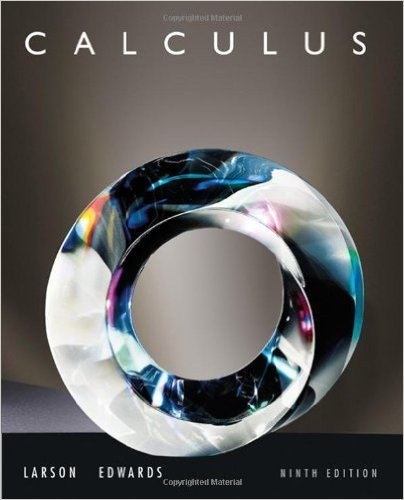×
×

# Solutions for Chapter 4.2: Area## Full solutions for Calculus | 9th Edition

ISBN: 9780547167022Solutions for Chapter 4.2: Area

Solutions for Chapter 4.2
4 5 0 398 Reviews
20
0
##### ISBN: 9780547167022

This expansive textbook survival guide covers the following chapters and their solutions. Since 93 problems in chapter 4.2: Area have been answered, more than 63720 students have viewed full step-by-step solutions from this chapter. Calculus was written by and is associated to the ISBN: 9780547167022. This textbook survival guide was created for the textbook: Calculus , edition: 9. Chapter 4.2: Area includes 93 full step-by-step solutions.

Key Calculus Terms and definitions covered in this textbook

P(A or B) = P(A) + P(B) - P(A and B). If A and B are mutually exclusive events, then P(A or B) = P(A) + P(B)

• Binomial coefficients

The numbers in Pascal’s triangle: nCr = anrb = n!r!1n - r2!

• Division algorithm for polynomials

Given ƒ(x), d(x) ? 0 there are unique polynomials q1x (quotient) and r1x(remainder) ƒ1x2 = d1x2q1x2 + r1x2 with with either r1x2 = 0 or degree of r(x) 6 degree of d1x2

• Even function

A function whose graph is symmetric about the y-axis for all x in the domain of ƒ.

• Exponential growth function

Growth modeled by ƒ(x) = a ? b a > 0, b > 1 .

• Heron’s formula

The area of ¢ABC with semiperimeter s is given by 2s1s - a21s - b21s - c2.

• Instantaneous velocity

The instantaneous rate of change of a position function with respect to time, p. 737.

• Interval

Connected subset of the real number line with at least two points, p. 4.

• Linear correlation

A scatter plot with points clustered along a line. Correlation is positive if the slope is positive and negative if the slope is negative

• Maximum r-value

The value of |r| at the point on the graph of a polar equation that has the maximum distance from the pole

• Midpoint (in a coordinate plane)

For the line segment with endpoints (a,b) and (c,d), (aa + c2 ,b + d2)

• Modulus

See Absolute value of a complex number.

• Negative association

A relationship between two variables in which higher values of one variable are generally associated with lower values of the other variable.

• One-to-one function

A function in which each element of the range corresponds to exactly one element in the domain

• Quotient of functions

a ƒ g b(x) = ƒ(x) g(x) , g(x) ? 0

• Replication

The principle of experimental design that minimizes the effects of chance variation by repeating the experiment multiple times.

• Rigid transformation

A transformation that leaves the basic shape of a graph unchanged.

• Rose curve

A graph of a polar equation or r = a cos nu.

• Spiral of Archimedes

The graph of the polar curve.

• Trigonometric form of a complex number

r(cos ? + i sin ?)

×